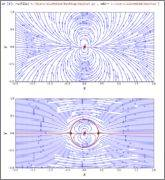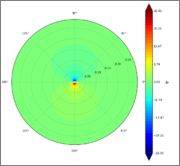# Doublet + Uniform Flow // Streamfunction Polar Plot Help

• Python
Hello,
Python,CFD and PF newbie here.
I am using Spyder (Python 3.5) and I managed to plot my streamfunction in cartesian coordinates.
I tried transforming and plotting in polar coordinates but I am not sure that what i have done is correct.
I am not even sure what the plot should look like :/
When I run the code the result is: [See attached picture]

The code is this:

Python:
import numpy
from matplotlib import pyplot
from matplotlib import cm

N=1000
r_min, r_max = 0,1
theta_min, theta_max = 0,2*numpy.pi
r=numpy.linspace(r_min,r_max,N)
theta=numpy.linspace(theta_min, theta_max,N)
X,Y=numpy.meshgrid(r*numpy.cos(theta),r*numpy.sin(theta))

kappa = 1
r_doublet, theta_doublet=0,0

def velocity_doublet(strength, rd, thetad, X, Y):

return u, v

def stream_function_doublet(strength, rd, thetad, X, Y):

return psi

# computes the velocity field on the mesh grid
u_doublet, v_doublet = velocity_doublet(kappa, r_doublet, theta_doublet, X, Y)

# computes the stream-function on the mesh grid
psi_doublet = stream_function_doublet(kappa, r_doublet, theta_doublet, X, Y)

#pyplot.streamplot(X, Y, u_doublet, v_doublet,
#density=2, linewidth=1, arrowsize=1, arrowstyle='->')
#pyplot.scatter(r_doublet, theta_doublet, color='#CD2305', s=80, marker='.');

#ax = pyplot.subplot(111, polar=True)
#ax.plot(X,Y, u_doublet,v_doublet, color='r', ls='none', marker='.')

u_inf = 1.0  # the speed of the freestream

u_freestream = u_inf * numpy.ones((N, N), dtype=float)
v_freestream = numpy.zeros((N, N), dtype=float)

psi_freestream = u_inf * Y

u = u_freestream + u_doublet
v = v_freestream + v_doublet
psi = psi_freestream + psi_doublet

ax = pyplot.subplot(111, polar=True)
pyplot.scatter(r_doublet*numpy.cos(theta_doublet), r_doublet*numpy.sin(theta_doublet), color='b', s=500, marker='o')
ax.contour(X,Y, psi, levels=[-1,1,N], colors='#CD2305',linestyles='solid')

#### Attachments

•PPlot.PNG
7.5 KB · Views: 759

jedishrfu
Mentor
Welcome to PF!

Have you tried plotting it in XY coordinates to compare it to your polar plot?

•nn2e11

Yes, everything worked fine in Cartesian coordinates. I cannot seem to find anything online either, as a reference plot at least.

Please see here for the X,Y. The upper plot is the doublet prior to applying the uniform flow. the bottom plot is the superposition of the two.Last edited by a moderator:
I think i got it right this time. In case someone finds it useful, I have attached the code below.

It produces the following polar plot which I think makes sense(?).Python:
import numpy
from matplotlib import pyplot
from matplotlib import cm

N=50
kappa = 1
r_doublet, theta_doublet=0,0
u_inf = 2.0  # the speed of the freestream
r_min, r_max = 0,1.025*numpy.sqrt(kappa/(2*numpy.pi*u_inf))
theta_min, theta_max = 0,2*numpy.pi
r=numpy.linspace(r_min,r_max,N)
theta=numpy.linspace(theta_min, theta_max,N)
X,Y=numpy.meshgrid(theta,r)

Q=numpy.sqrt(strength/(2*numpy.pi*u_inf))

return V_r, V_theta

Q=numpy.sqrt(strength/(2*numpy.pi*u_inf))

return psi

# computes the velocity field on the mesh grid
u_doublet, v_doublet = velocity_doublet(kappa, r_doublet, theta_doublet, X,Y)

# computes the stream-function on the mesh grid
psi_doublet = stream_function_doublet(kappa, r_doublet, theta_doublet, X,Y)
pyplot.figure(figsize=(10, 10))
ax=pyplot.subplot(111, polar=True)
ax.set_rmax(r_max)
ax.set_rmin(r_min)
ax.grid(True)
minpsi=numpy.nanmin(psi_doublet)
maxpsi=numpy.nanmax(psi_doublet)
avgpsi=(minpsi+maxpsi)*0.5
ax.contourf(X,Y, psi_doublet, levels=numpy.linspace(numpy.nanmin(psi_doublet), numpy.nanmax(psi_doublet),N),cmap=cm.Spectral)
contf = pyplot.contourf(X, Y, psi_doublet, levels=numpy.linspace(minpsi, maxpsi, N), extend='both',cmap=cm.jet)
cbar = pyplot.colorbar(contf)
cbar.set_label('$Ψ$', fontsize=16)
cbar.set_ticks([minpsi,0.75*minpsi,0.5*minpsi,0.25*minpsi,avgpsi,0.25*maxpsi,0.5*maxpsi,0.75*maxpsi, maxpsi])

Last edited by a moderator:
•Pepper Mint